# MCQs on Electric Circuits

##### Page 62 of 63. Go to page 1 2 3 4 5 6 7 8 9 10 11 12 13 14 15 16 17 18 19 20 21 22 23 24 25 26 27 28 29 30 31 32 33 34 35 36 37 38 39 40 41 42 43 44 45 46 47 48 49 50 51 52 53 54 55 56 57 58 59 60 61 62 63
01․ In the circuit shown below, find the thevnin equivalent resistance.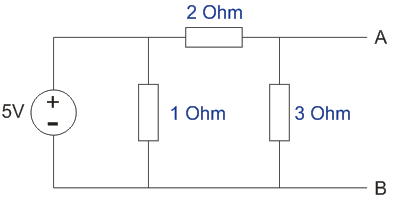6/5 Ohm.
5/6 Ohm.
5 Ohm.
1.5 Ohm.

To find the thevnin resistance, the voltage source is short circuited and the equivalent resistance looking into the circuit from AB terminals is 3||2 = 6/5 Ohm as no current will flow through 1 Ohm resistance.

02․ Two electric bulbs have transparent filament of same thickness. It one of them gives 60 W and other gives 100 W, then-
60 W lamp filament has shorter length.
100 W lamp filament has longer length.
60 W lamp filament has longer length.
60 W and 100 W lamp filament have equal length.

We know,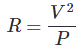So, higher wattage means lower resistance. Resistance of 60 Watt lamp is greater than 100 Watt lamp Now,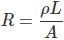So, which has higher resistance it has longer length.

03․ Bulb in street lighting are connected in
parallel.
series.
series parallel.
end of end.

In parallel connection voltage drop across each electrical apparatus get some voltage i.e. 200v. It any of them fuse out, the remaining circuit becomes unaltered.

04․ From the circuit diagram shown in question 1 find the thevnin voltage.
5 V.
15 V.
1.5 V.
3 V.

To find the thevnin voltage, total current in the circuit is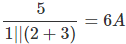The current through the 3 Ohm resistance is 6 × 1/(1+2+3) = 1A; So open source voltage across the terminals is 3 × 1 = 3V.

05․ From the circuit diagram shown in question 1 find the Norton current.
2 A.
2.5 A.
4 A.
1 A.

To find the Norton current, the terminals AB are short circuited and the current bypasses the 3 Ohm resistance. Total current is given by 5/(2|| 1) = 7.5 A. So the current through the short circuited AB terminals is 7.5 × 1/(1+2) = 2.5 A.

06․ Five cells are connected in series in a row and then four such rows are connected in parallel to Feed the current to a resistive load of 1.25Ω. Each cell has an emf of 1.5 V with internal resistance Of 0.2Ω. The current through the load will be
3.33 A
23.33 A
5 A
1 A

Five cells are connected in series and each has emfof 1.5 V and resistance of 0.2 ? and four such rows are connected in parallel to feed the current to a resistive load of 1.25?. So total emf is 1.5 × 5 = 7.5 V Total equivalent resistance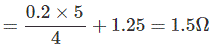Hence the current through the load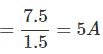07․ If the measured value of a capacitor is 205.3µF and true value is 201.4µF the percentage relative error is
1.94%
25.64%
1.89%
39.00%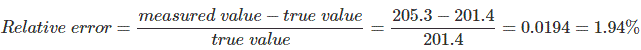08․ The efficiency in case of maximum power transfer theorem is
100 %.
less than 50 %.
50 %.
50 % - 100 %.

Maximum power is transferred when the internal resistance is equal to load resistance. So, the source power is distributed equally between the source internal impedance and the load impedance; i.e. the maximum efficiency is 50%.

09․ From the circuit diagram shown in question 1 find the maximum power transferred to the load.
1.875 W.
4.547 W.
9 W.
15.247 W.

Maximum power transfer will take place when the load resistance is equal to thevnin resistance and is given by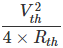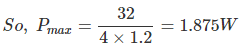10․ A piece of copper and another piece of germanium are cooled from room temperature to 80K. The resistance of
each of them increases.
each of them decreases.
copper increases and germanium decreases.
copper decreases and germanium increases.

Copper is a metal and it has positive temperature co-efficient. Germanium is a semiconductor and it has negative temperature co-efficient. So when the temperature decreases the resistance of copper decreases and resistance of germanium increases.

<<<60616263>>>